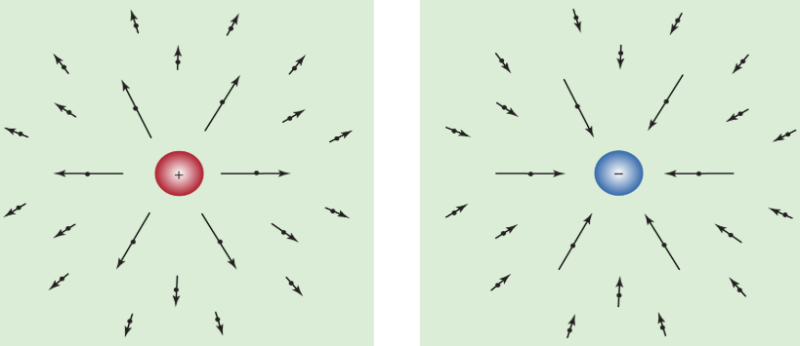Courses

# Electric fields and forces Physics Notes | EduRev

## Physics : Electric fields and forces Physics Notes | EduRev

The document Electric fields and forces Physics Notes | EduRev is a part of the Physics Course Basic Physics for IIT JAM.
All you need of Physics at this link: Physics

The force and conservation laws are only two aspects of electromagnetism, however. Electric and magnetic forces are caused by electromagnetic fields. The term field denotes a property of space, so that the field quantity has a numerical value at each point of space. These values may also vary with time. The value of the electric or magnetic field is a vector—i.e., a quantity having both magnitude and direction. The value of the electric field at a point in space, for example, equals the force that would be exerted on a unit charge at that position in space.
Every charged object sets up an electric field in the surrounding space. A second charge “feels” the presence of this field. The second charge is either attracted toward the initial charge or repelled from it, depending on the signs of the charges. Of course, since the second charge also has an electric field, the first charge feels its presence and is either attracted or repelled by the second charge too.
The electric field from a charge is directed away from the charge when the charge is positive and toward the charge when it is negative. The electric field from a charge at rest is shown in Figure 1 for various locations in space. The arrows point in the direction of the electric field, and the length of the arrows indicates the strength of the field at the midpoint of the arrows.Figure 1: Electric fields. (Left) Field of a positive electric charge and (right) field of a negative electric charge.

If a positive charge were placed in the electric field, it would feel a force in the direction of the field. A negative charge would feel a force in the direction opposite the direction of the field.
In calculations, it is often more convenient to deal directly with the electric field than with the charges. Frequently, more is known about the field than about the distribution of charges in space. For example, the distribution of charges in conductors is generally unknown because the charges move freely within the conductor. In static situations, however, the electric field in a conductor in equilibrium has a definite value, zero, because any force on the charges inside the conductor redistributes them until the field vanishes. The unit of electric field is newtons per coulomb, or volts per metre.
The electric potential is another useful field. It provides an alternative to the electric field in electrostatics problems. The potential is easier to use, however, because it is a single number, a scalar, instead of a vector. The difference in potential between two places measures the degree to which charges are influenced to move from one place to another. If the potential is the same at two places (i.e., if the places have the same voltage), charges will not be influenced to move from one place to the other. The potential on an object or at some point in space is measured in volts; it equals the electrostatic energy that a unit charge would have at that position. In a typical 12-volt car battery, the battery terminal that is marked with a + sign is at a potential 12 volts greater than the potential of the terminal marked with the − sign. When a wire, such as the filament of a car headlight, is connected between the + and the − terminals of the battery, charges move through the filament as an electric current and heat the filament, and the hot filament radiates light.

Offer running on EduRev: Apply code STAYHOME200 to get INR 200 off on our premium plan EduRev Infinity!

## Basic Physics for IIT JAM

109 videos|157 docs|94 tests

,

,

,

,

,

,

,

,

,

,

,

,

,

,

,

,

,

,

,

,

,

;